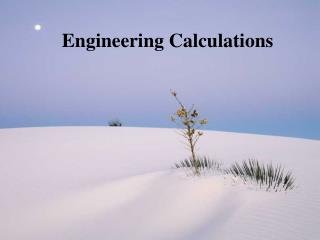DownloadDownload PresentationEngineering Calculations

# Engineering Calculations

Télécharger la présentation## Engineering Calculations

- - - - - - - - - - - - - - - - - - - - - - - - - - - E N D - - - - - - - - - - - - - - - - - - - - - - - - - - -
##### Presentation Transcript

1. Engineering Calculations

2. Engineering Dimensions and Units Density D = M/V M = mass V = volume Water: 1 x 103 kg/m3 or 1 g/cm3 or 62.4 lbm/ft3

3. Concentration CA = concentration of A MA = mass of material A VA = volume of material A VB = volume of material B

4. Concentration Parts per million is a common measure of Concentration (ppm). It is equivalent to mg/L in water since one milliliter of water weighs one gram.

5. Mass Concentration

6. Concentration 10,000 mg/L = 10,000 ppm (if D = 1) 10,000 ppm = 1% (by weight)

7. Flow Rate Volumetric flow rate m3/s , ft3/s, gpm, mgd Mass (gravimetric) flow rate kg/s, lbm/s Realted since mass = density x volume (QM = QVD)

8. Mass flow of a component Qma = CA x QV(A+B) Example: A wastewater treatment plant discharges a flow of 1.5 m3/s at a solids concentration of 20 mg/L. How much solids is the plant discharging each day? Qma= 20 mg/L X (1 x 10-6 kg/mg) x (1.5 m3/s x 103 L/m3 x 86,400 s/day) = 2592 kg/day

9. A useful conversion Volumetric flow rate in million gallons per day, mgd Mass flow rate in pound per day Concentration in mg/L = x x 8.34

10. Residence Time Also referred to as detention time or retention time The average amount of time it takes a particle of fluid spends in a container it is flowing through or you can think of it as the amount of time it takes to fill the container. - t = V/Q Example: A lagoon has a volume of 1500 m3 amd the flow into the lagoon is 3 m3/hour. What is the residence time in this lagoon? - t = 1500/3 = 500 hours

11. Approximations in Calculations • Define the problem • Introduce simplifying assumptions • Calculate answer • 4. Check the answer

12. Significant Figures Significant figures are those that transfer information based on the value of the digit